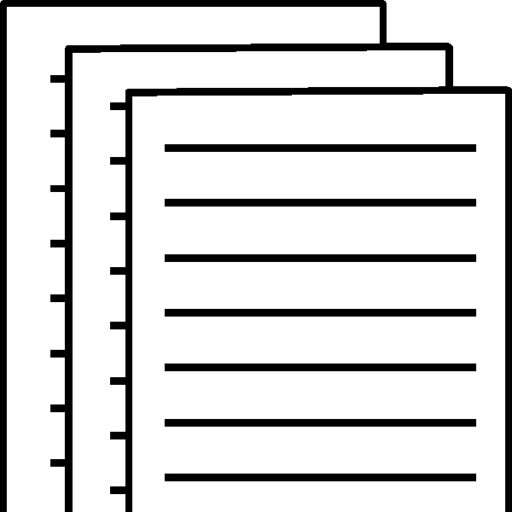## ACC 557 Final Exam Part 2

ACC 557 Final Exam Part 2

Question 1

3 out of 3 points

 The cost method of accounting for long-term investments in stock should be employed when the Selected Answer: investor’s influence on the investee is insignificant.Correct Answer: investor’s influence on the investee is insignificant.

Question 2

0 out of 3 points

 The cost of debt investments includes each of the following except Selected Answer: commissions.Correct Answer: accrued interest.

Question 3

3 out of 3 points

 Comanic Corp. has common stock of \$5,400,000, retained earnings of \$2,000,000, unrealized gains on trading securities of \$100,000 and unrealized losses on available-for-sale securities of \$200,000.  What is the total amount of its stockholders’ equity? Selected Answer: \$7,200,000Correct Answer: \$7,200,000

Question 4

3 out of 3 points

 Corporations invest excess cash for short periods of time in each of the following except Selected Answer: equity securities.Correct Answer: equity securities.

Question 5

3 out of 3 points

 Revenue is recognized when cash dividends are received under Selected Answer: the cost method.Correct Answer: the cost method.

Question 6

3 out of 3 points

 Pension funds and mutual funds regularly invest in debt and stock securities to Selected Answer: generate earnings.Correct Answer: generate earnings.

Question 7

3 out of 3 points

 Unrealized gains and losses related to available-for-sale/non-trading equity investments are reported in other comprehensive income under Selected Answer: Both GAAP and IFRS.Correct Answer: Both GAAP and IFRS.

Question 8

3 out of 3 points

 If a parent company has two wholly owned subsidiaries, how many legal and economic entities are there from the viewpoint of the shareholders of the parent company?        Legal             Economic Selected Answer: 3                        1Correct Answer: 3                        1

Question 9

3 out of 3 points

 Comparisons of financial data made within a company are called Selected Answer: intracompany comparisons.Correct Answer: intracompany comparisons.

Question 10

3 out of 3 points

 Under IFRS, comprehensive income may be displayed (reported) in Selected Answer: the one-statement or the two-statement approach.Correct Answer: the one-statement or the two-statement approach.

Question 11

3 out of 3 points

 Each of the following is a factor affecting quality of earnings except Selected Answer: extraordinary items.Correct Answer: extraordinary items.

Question 12

3 out of 3 points

 Wrapp Company has income before taxes of \$350,000 and an extraordinary loss of \$70,000. If the income tax rate is 30% on all items, the income statement should show income before irregular items and an extraordinary loss, respectively, of: Selected Answer: \$245,000 and (\$49,000)Correct Answer: \$245,000 and (\$49,000)

Question 13

3 out of 3 points

 Vertical analysis is a technique which expresses each item within a financial statement Selected Answer: in terms of a percent of a base amount.Correct Answer: in terms of a percent of a base amount.

Question 14

3 out of 3 points

 Swiss Clothing Store had a balance in the Accounts Receivable account of \$920,000 at the beginning of the year and a balance of \$980,000 at the end of the year. Net credit sales during the year amounted to \$6,650,000. The average collection period of the receivables in terms of days was Selected Answer: 52.1 days.Correct Answer: 52.1 days.

Question 15

3 out of 3 points

 Under which of the following cases may a percentage change be computed? Selected Answer: The trend of the balances is decreasing but all balances are positive.Correct Answer: The trend of the balances is decreasing but all balances are positive.

Question 16

3 out of 3 points

 Which one of the following is not a tool in financial statement analysis? Selected Answer: Circular analysisCorrect Answer: Circular analysis

Question 17

3 out of 3 points

 The following information pertains to Rural Company. Assume that all balance sheet amounts represent both average and ending balance figures.  Assume that all sales were on credit.AssetsCash and short-term investments                                                                                   \$  40,500Accounts receivable (net)                                                                                                     30,000Inventory                                                                                                                                  27,000Property, plant and equipment                                                                                         215,000               Total Assets                                                                                                          \$312,500Liabilities and Stockholders’ EquityCurrent liabilities                                                                                                                \$  60,000Long-term liabilities                                                                                                               75,000Stockholders’ equity—common                                                                                        177,500               Total Liabilities and Stockholders’ Equity                                                              \$312,500Income StatementSales                                                                                                                                      \$  90,000Cost of goods sold                                                                                                                 40,000Gross profit                                                                                                                              50,000Operating expenses                                                                                                               25,000               Net income                                                                                                                  \$  25,000Number of shares of common stock                                                                                    5,000Market price of common stock                                                                                                 \$22Dividends per share                                                                                                                    1.00 What is the return on assets for Rural? Selected Answer: 8%Correct Answer: 8%

Question 18

0 out of 3 points

 Which of the following transactions does notaffect cash during a period? Selected Answer: Exercise of the call option on bonds payableCorrect Answer: Write-off of an uncollectible account

Question 19

3 out of 3 points

 Jean’s Vegetable Market had the following transactions during 2014:1. Issued \$50,000 of par value common stock for cash.2. Repaid a 6 year note payable in the amount of \$22,000. 3. Acquired land by issuing common stock of par value \$100,000.4. Declared and paid a cash dividend of \$2,000. 5. Sold a long-term investment (cost \$3,000) for cash of \$8,000. 6. Acquired an investment in IBM stock for cash of \$15,000. What is the net cash provided (used) by investing activities? Selected Answer: (\$7,000)Correct Answer: (\$7,000)

Question 20

3 out of 3 points

 Which of the following would be added to net income using the indirect method? Selected Answer: Depreciation expenseCorrect Answer: Depreciation expense

Question 21

3 out of 3 points

 The order of presentation of activities on the statement of cash flows is Selected Answer: operating, investing, and financing.Correct Answer: operating, investing, and financing.

Question 22

3 out of 3 points

 In calculating cash flows from operating activities using the indirect method, a loss on the sale of equipment will appear as a(n) Selected Answer: addition to net income.Correct Answer: addition to net income.

Question 23

3 out of 3 points

 A company had net income of \$210,000. Depreciation expense is \$27,000. During the year, Accounts Receivable and Inventory increased \$17,000 and \$42,000, respectively. Prepaid Expenses and Accounts Payable decreased \$5,000 and \$6,000, respectively. There was also a loss on the sale of equipment of \$2,000. How much cash was provided by operating activities? Selected Answer: \$179,000Correct Answer: \$179,000

Question 24

3 out of 3 points

 Which of the following activities is excluded from the statement of cash flows under IFRS? Selected Answer: Noncash investing and financing activitiesCorrect Answer: Noncash investing and financing activities

Question 25

3 out of 3 points

 Which one of the following affects cash during a period? Selected Answer: Payment of an accounts payableCorrect Answer: Payment of an accounts payable

To view and download a complete answer, scroll down to the bottom to pay